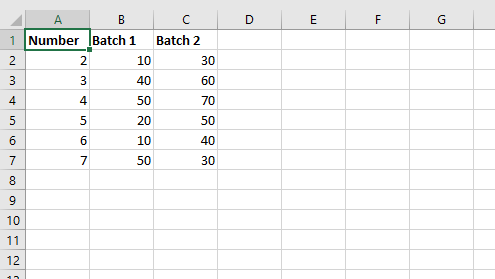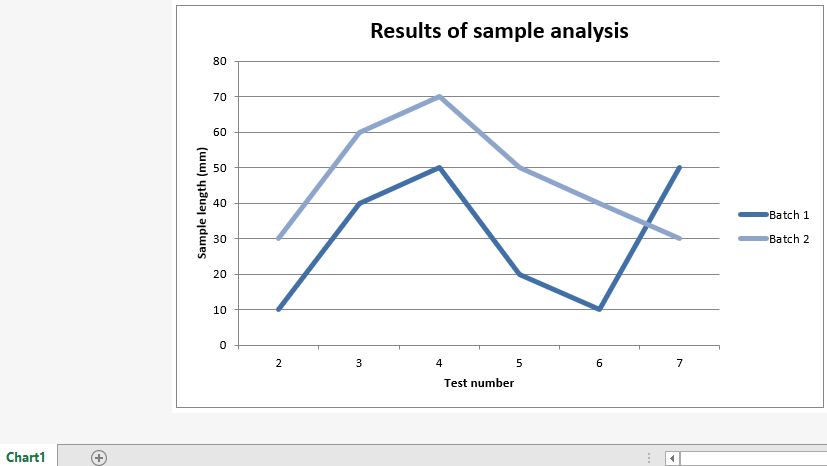GeeksforGeeks App
Open AppBrowser
Continue

# Python | Adding a Chartsheet in an excel sheet using XlsxWriter module

XlsxWriter is a Python library using which one can perform multiple operations on excel files like creating, writing, arithmetic operations, and plotting graphs. Let’s see how to add a chartsheet to an excel sheet and plot a line chart on it, using real-time data. Charts are composed of at least one series of one or more data points. Series themselves are comprised of references to cell ranges. For plotting the charts on a chartsheet, firstly, create a chart object of a specific chart type(i.e Line chart, etc). After creating chart objects, insert data in it, and lastly, add that chart object to the chartsheet object.

Prerequisite: Create and Write on an excel sheet

Code: Adding the chartsheet in an excel sheet. For creating a worksheet that only holds a chart we are using add_chartsheet() method of workbook object.

## Python3

 `# Python3 program for adding a chartsheet``# in an excel sheet using xlsxwriter` `# we import xlsxwriter module``import` `xlsxwriter` `# Workbook() takes one, non-optional, argument``# which is the filename that we want to create.``workbook ``=` `xlsxwriter.Workbook(``'chartsheet.xlsx'``)` `# The workbook object is then used to add new``# worksheet via the add_worksheet() method.``worksheet ``=` `workbook.add_worksheet()` `# create a worksheet that only holds a chart``# using add_chartsheet() method of workbook object.``chartsheet ``=` `workbook.add_chartsheet()` `# Create a new Format object to formats cells``# in worksheets using add_format() method .` `# here we create bold format object``bold ``=` `workbook.add_format({``'bold'``: ``1``})` `# Add the worksheet data that the charts will refer to.``headings ``=` `[``'Number'``, ``'Batch 1'``, ``'Batch 2'``]``data ``=` `[``    ``[``2``, ``3``, ``4``, ``5``, ``6``, ``7``],``    ``[``10``, ``40``, ``50``, ``20``, ``10``, ``50``],``    ``[``30``, ``60``, ``70``, ``50``, ``40``, ``30``],``]` `# Write a row of data starting from 'A1'``# with bold format .``worksheet.write_row(``'A1'``, headings, bold)` `# Write a column of data starting from``# 'A2', 'B2', 'C2' respectively .``worksheet.write_column(``'A2'``, data[``0``])``worksheet.write_column(``'B2'``, data[``1``])``worksheet.write_column(``'C2'``, data[``2``])`  `# Create a chart object that can be added``# to a worksheet using add_chart() method.` `# here we create a line chart object .``chart1 ``=` `workbook.add_chart({``'type'``: ``'line'``})` `# Add a data series to a chart``# using add_series method.` `# Configure the first series.``# = Sheet1 !\$A\$1 is equivalent to ['Sheet1', 0, 0].` `# note : spaces is not inserted in b / w``# = and Sheet1, Sheet1 and !``# if space is inserted it throws warning.``chart1.add_series({``    ``'name'``:       ``'= Sheet1 !\$B\$1'``,``    ``'categories'``: ``'= Sheet1 !\$A\$2:\$A\$7'``,``    ``'values'``:     ``'= Sheet1 !\$B\$2:\$B\$7'``, })`  `# Configure a second series.``# Note use of alternative syntax to define ranges.``# [sheetname, first_row, first_col, last_row, last_col].``chart1.add_series({``    ``'name'``:       [``'Sheet1'``, ``0``, ``2``],``    ``'categories'``: [``'Sheet1'``, ``1``, ``0``, ``6``, ``0``],``    ``'values'``:     [``'Sheet1'``, ``1``, ``2``, ``6``, ``2``], })` `# Add a chart title``chart1.set_title({``'name'``: ``'Results of sample analysis'``})` `# Add x-axis label``chart1.set_x_axis({``'name'``: ``'Test number'``})` `# Add y-axis label``chart1.set_y_axis({``'name'``: ``'Sample length (mm)'``})` `# Set an Excel chart style.``chart1.set_style(``11``)` `# Add the chart to the chartsheet.``# chartsheet.set_chart(chart1)``chartsheet.insert_chart(``'B3'``, chart1)` `# Display the chartsheet as the``# active sheet when the workbook is opened.``chartsheet.activate()` `# Finally, close the Excel file``# via the close() method.``workbook.close()`

Output:My Personal Notes arrow_drop_up